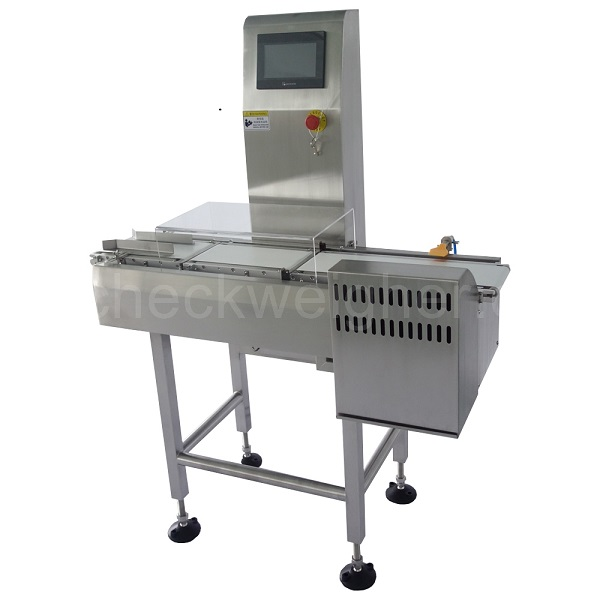# 중량 저울 확인을위한 두 가지 정밀 테스트 방법

Check weighing scale(also called checkweigher,weighing scale and sorting machine) is a key equipment, automatic production line is very it has high precision, high speed, can save a single product weight and total weight per batch products, and other functions, but in the use of inspection in the process of weighing scales, want to ensure that every batch of products can achieve accurate measurement, it needs to check to test the accuracy of weighing scales, and precise test that how to correct?The following small series of Shanghai practical for you to collect two test methods, I hope to help you.The first test is to pass the same product 10 or 20 times, making sure that each pass is in the same direction as you would in production.1. Record each data. Some check scales can automatically record and calculate standard deviation.If the balance cannot be calculated automatically, it can be calculated using the STDEV formula in Excel.2. After the standard deviation, multiply by 6 to obtain the unconfidence interval (a calculation method for the uncertainty of dynamic weight detection globally accepted).3. Retrieve the TNE(-T1) in the table below. For example, if your product weighs 200 grams, the TNE(-T1) is 5% or 9g.4. Now calculate a quarter of this 9g, which is 0.25 x 9g = 2.25g.This is your limit for repeatability.5. If your initial unconfidence interval is greater than 2.25g, then the weigher is not available under this setting.This test is required for all different types and weights of products to be checked on the weigher.To do this better, repeat the test every once in a while, recording and analyzing trends for each test to see how the results change over time.Based on this trend, you can determine how often you need to run the test.These records, along with trend analysis, are also valid evidence of the property risk assessment of the weigher users.
The second test is also carried out using the product under test. In addition, a calibrated static balance is also needed.The resolution of the static balance should be the same as that of the check weigher, or higher.This inspection is required before, after, and at the end of a batch.Again, this test can do trend analysis for further risk assessment.1. Use correction weights of similar weight to the product to check whether the static scale can be weighed correctly.2. Pass the running check weigher, and then put it on the static scale for weighing.Compare the weight value on the checking scale with that on the static scale.If the difference is greater than 1/5 of the TNE, this check weigher is not applicable.When the batch is in progress, some checkweighers have a built-in weight check function, which removes the product for testing only and displays the weight on the screen at a constant rate for testing purposes.3. Repeat this test at least once to check the repeatability of the weigher.예를 들어, 당사 제품의 정격 중량이 200g 인 경우 계량 저울의 승인 된 중량은 200.4g이고 동일한 제품을 정적 저울에 올려 놓고 무게를 측정하면 199.7g으로 표시되며 차이는 0.7g입니다. 200g 제품의 TNE는 9g, 5 분의 1은 1.8g, 0.7g이 1.8g 미만일 때 체크 계량기를 정확하게 측정하여 정상적으로 사용할 수 있습니다.제품 테스트의 각 배치가 정확하려면이 두 가지 테스트를 실행하고 표준 워크 플로에 작성해야합니다. 일정 시간 후에 두 테스트를 반복하고 테스트를 통과하면 계량기를 안전하게 사용할 수 있습니다.

이 두 가지 방법이 도움을 줄 수 있기를 바랍니다. 여전히 내용을 이해하려면 여기를 클릭하여 상담하십시오 !
Chat with us